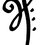# HOOKE'S LAW

Hooke’s Law is a law that shows the relationship between the forces applied to a spring and its elasticity. The relationship is best explained by the equation F=-kx. F is force applied to the spring this can be either the strain or stress that acts upon the spring. X is the displacement of the spring with negative value demonstrating that the displacement of the spring when it is stretched. When the spring is compressed the the x value is positive. K is the spring constant and details how stiff the spring is. This law gets its name from the Robert Hooke the 17th century physicist who discovered it in 1660 and published a work that included a description of it in 1678.

The law had many important practical applications with one being the creation of a balance spring that made the creation of a portable timepiece possible. Hooke’s law is the first classical example of an explanation of elasticity. So you can thank Hooke’s law for the wristwatch that you wear or the alarm clock next to your bed.

Another interesting thing about Hooke’s law is that it is a a perfect example of the First Law of Thermodynamics. Any spring when compressed or extended almost perfectly conserved the energy applied to it. The only energy lost is due to natural friction.

Like most classical mechanics Hooke’s Law only works in a limited frame of reference. In this case, it is that the law is only most effective for relatively small deformations in the spring. Anything greater would need another method to be explained mathematically.Hooke’s law is just the basic understanding of elasticity. There are other principles that explain this for other substancesNote by Vishwathiga Jayasankar
6 years, 9 months ago

This discussion board is a place to discuss our Daily Challenges and the math and science related to those challenges. Explanations are more than just a solution — they should explain the steps and thinking strategies that you used to obtain the solution. Comments should further the discussion of math and science.

When posting on Brilliant:

• Use the emojis to react to an explanation, whether you're congratulating a job well done , or just really confused .
• Ask specific questions about the challenge or the steps in somebody's explanation. Well-posed questions can add a lot to the discussion, but posting "I don't understand!" doesn't help anyone.
• Try to contribute something new to the discussion, whether it is an extension, generalization or other idea related to the challenge.

MarkdownAppears as
*italics* or _italics_ italics
**bold** or __bold__ bold
- bulleted- list
• bulleted
• list
1. numbered2. list
1. numbered
2. list
Note: you must add a full line of space before and after lists for them to show up correctly
paragraph 1paragraph 2

paragraph 1

paragraph 2

[example link](https://brilliant.org)example link
> This is a quote
This is a quote
    # I indented these lines
# 4 spaces, and now they show
# up as a code block.

print "hello world"
# I indented these lines
# 4 spaces, and now they show
# up as a code block.

print "hello world"
MathAppears as
Remember to wrap math in $$ ... $$ or $ ... $ to ensure proper formatting.
2 \times 3 $2 \times 3$
2^{34} $2^{34}$
a_{i-1} $a_{i-1}$
\frac{2}{3} $\frac{2}{3}$
\sqrt{2} $\sqrt{2}$
\sum_{i=1}^3 $\sum_{i=1}^3$
\sin \theta $\sin \theta$
\boxed{123} $\boxed{123}$